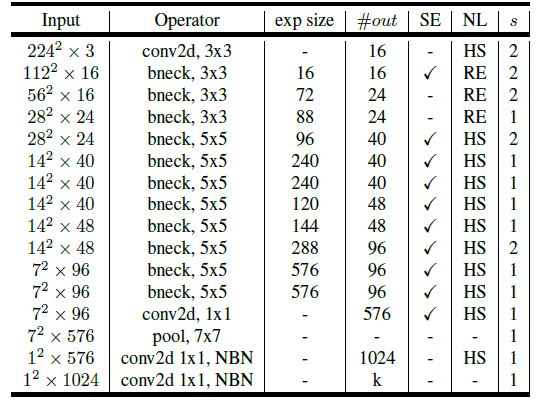×

Search anything:

# MobileNet V1 Architecture

#### Machine Learning (ML)Get this book -> Problems on Array: For Interviews and Competitive Programming

MobileNet V1 is a variant of MobileNet model which is specially designed for edge devices. We have explored the MobileNet V1 architecture in depth.

Convolutional Neural Networks(CNN) have become very popular in computer vision. However, in order to achieve a higher degree of accuracy modern CNNs are becoming deeper and increasingly complex. Such networks cannot be used in real applications like robots and self driving cars.In this section we will be discussing a CNN architecture that aims to effectively tackle this problem.

# MobileNet

MobileNet is an efficient and portable CNN architecture that is used in real world applications. MobileNets primarily use depthwise seperable convolutions in place of the standard convolutions used in earlier architectures to build lighter models.MobileNets introduce two new global hyperparameters(width multiplier and resolution multiplier) that allow model developers to trade off latency or accuracy for speed and low size depending on their requirements.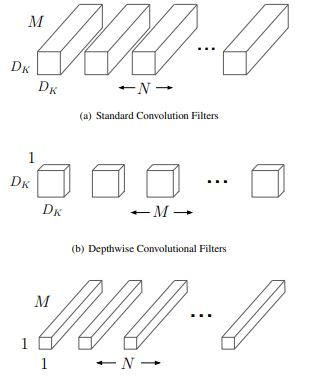# Architecture

MobileNets are built on depthwise seperable convolution layers.Each depthwise seperable convolution layer consists of a depthwise convolution and a pointwise convolution.Counting depthwise and pointwise convolutions as seperate layers, a MobileNet has 28 layers.A standard MobileNet has 4.2 million parameters which can be further reduced by tuning the width multiplier hyperparameter appropriately.
The size of the input image is 224 × 224 × 3.

The detailed architecture of a MobileNet is given below :

Type Stride Kernel Shape Input Size
Conv 2 3 × 3 × 3 × 32 224 × 224 × 3
Conv dw 1 3 × 3 × 32 112 × 112 × 32
Conv 1 1 × 1 × 32 × 64 112 × 112 × 32
Conv dw 2 3 × 3 × 64 112 × 112 × 64
Conv 1 1 × 1 × 64 × 128 56 × 56 × 64
Conv dw 1 3 × 3 × 128 56 × 56 × 128
Conv 1 1 × 1 × 128 × 128 56 × 56 × 128
Conv dw 2 3 × 3 × 128 56 × 56 × 128
Conv 1 1 × 1 × 128 × 256 56 × 56 × 128
Conv dw 1 3 × 3 × 256 28 × 28 × 256
Conv 1 1 × 1 × 256 × 256 28 × 28 × 256
Conv dw 2 3 × 3 × 256 28 × 28 × 256
Conv 1 1 × 1 × 256 × 512 14 × 14 × 256
Conv dw 1 3 × 3 × 512 14 × 14 × 512
Conv 1 1 × 1 × 512 × 512 14 × 14 × 512
Conv dw 1 3 × 3 × 512 14 × 14 × 512
Conv 1 1 × 1 × 512 × 512 14 × 14 × 512
Conv dw 1 3 × 3 × 512 14 × 14 × 512
Conv 1 1 × 1 × 512 × 512 14 × 14 × 512
Conv dw 1 3 × 3 × 512 14 × 14 × 512
Conv 1 1 × 1 × 512 × 512 14 × 14 × 512
Conv dw 1 3 × 3 × 512 14 × 14 × 512
Conv 1 1 × 1 × 512 × 512 14 × 14 × 512
Conv dw 2 3 × 3 × 512 14 × 14 × 512
Conv 1 1 × 1 × 512 × 1024 7 × 7 × 512
Conv dw 2 3 × 3 × 1024 7 × 7 × 1024
Conv 1 1 × 1 × 1024 × 1024 7 × 7 × 1024
Avg Pool 1 Pool 7 × 7 7 × 7 × 1024
Fully Connected 1 1024 × 1000 1 × 1 × 1024
Softmax 1 Classifier 1 × 1 × 1000

Standard Convoltion layer :
A single standard convolution unit (denoted by Conv in the table above) looks like this :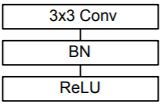Depthwise seperable Convolution layer
A single Depthwise seperable convolution unit (denoted by Conv dw in the table above) looks like this :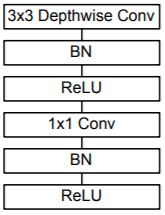Width Multiplier
Width muliplier (denoted by α) is a global hyperparameter that is used to construct smaller and less computionally expensive models.Its value lies between 0 and 1.For a given layer and value of α, the number of input channels 'M' becomes α * M and the number of output channels 'N' becomes α * N hence reducing the cost of computation and size of the model at the cost of performance.The computation cost and number of parameters decrease roughly by a factor of α2.Some commonly used values of α are 1,0.75,0.5,0.25.

Resolution Multiplier
The second parameter introduced in MobileNets is called resolution multiplier and is denoted by ρ.This hyperparameter is used to decrease the resolution of the input image and this subsequently reduces the input to every layer by the same factor. For a given value of ρ the resolution of the input image becomes 224 * ρ.This reduces the computational cost by a factor of ρ2.

# MobileNetV2

A few significant changes were made to the MobileNetV1 architecture that resulted in a considerable increase in the accuracy of the model.The main changes made to the architecture were the introduction of inverted residual blocks and linear bottlenecks and the use of the ReLU6 activation function in place of ReLU.
The architecture of the MobileNetV2 is given below :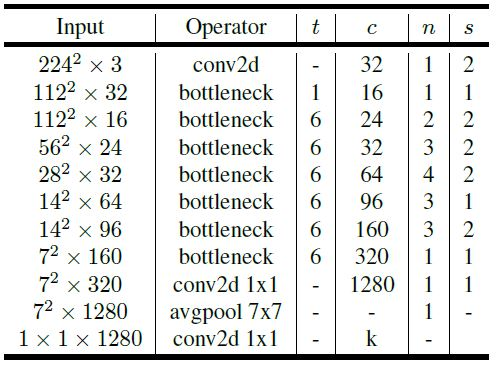'c' is the number of output channels(or number of filters)
't' is the expansion factor
'n' is the number of times the block is repeated
's' is the stride

# MobileNetV3

There are two different MobileNetV3 architectures :

• MobileNetV3 small
• MobileNetV3 large

The MobileNetV3 small is 6.6% more accurate on the ImageNet classification than MobileNetV2 and has similar latency.The MobileNetV3 large is 3.2% more accurate on ImageNet classification and reduces latency by 20% when compared to the MobileNetV2.

MobileNetV3 large :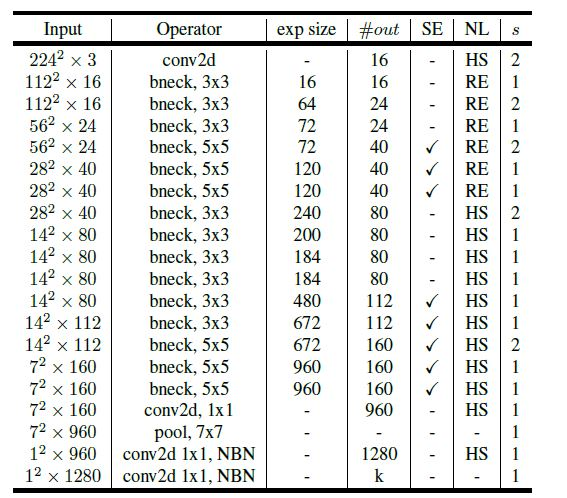NBN denotes No Batch Normalization
NL denotes Non Linearity
HS denotes h-swish
RE denotes ReLU

MobileNetV3 small :